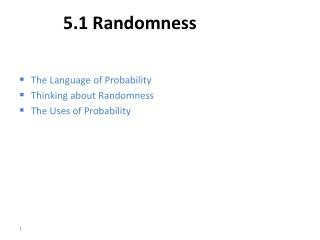Download Presentation5.1 Randomness

# 5.1 Randomness - PowerPoint PPT PresentationDownload Presentation## 5.1 Randomness

- - - - - - - - - - - - - - - - - - - - - - - - - - - E N D - - - - - - - - - - - - - - - - - - - - - - - - - - -
##### Presentation Transcript

1. 5.1 Randomness • The Language of Probability • Thinking about Randomness • The Uses of Probability

2. The Language of Probability Chance behavior is unpredictable in the short run, but has a regular and predictable pattern in the long run. We call a phenomenon randomif individual outcomes are uncertain but there is nonetheless a regular distribution of outcomes in a large number of repetitions. The probabilityof any outcome of a chance process is the proportion of times the outcome would occur in a very long series of repetitions – relative frequency!

3. Thinking about Randomness The result of any single coin toss is random. But the result over many tosses is predictable, as long as the trials are independent (i.e., the outcome of a new coin flip is not influenced by the result of the previous flip). The probability of heads is 0.5 = the proportion of times you get heads in many repeated trials. First series of tosses Second series

4. 5.2 Probability Models • Sample Spaces • Probability Rules • Assigning Probabilities • Independence and the Multiplication Rule

5. Probability Models Descriptions of chance behavior contain two parts: a list of possible outcomes and a probability for each outcome. The sample space Sof a chance process is the set of all possible outcomes. An eventis an outcome or a set of outcomes of a random phenomenon. That is, an event is a subset of the sample space. A probability modelis a description of some chance process that consists of two parts: a sample space S and a probability for each outcome.

6. Probability Models Example: Give a probability model for the chance process of rolling two fair, six-sided dice―one that’s red and one that’s green. Sample Space 36 Outcomes Since the dice are fair, each outcome is equally likely. Each outcome has probability 1/36.

7. Probability Rules Any probability is a number between 0 and 1. All possible outcomes taken together must sum to probability 1. If two events have no outcomes in common, the probability that one or the other occurs is the sum of their individual probabilities. The probability that an event does not occur is 1 minus the probability that the event does occur. Rule 1. The probability P(A) of any event A satisfies 0 ≤ P(A) ≤ 1. Rule 2. If S is the sample space in a probability model, then P(S) = 1. Rule 3. If A and B are disjoint,P(A or B) = P(A) + P(B). This is the addition rule for disjoint events. Rule 4:The complement of any event A is the event that A does not occur, written AC. P(AC) = 1 – P(A).

8. Venn Diagrams Two events that are not disjoint, and the event {A and B} consisting of the outcomes they have in common: Two disjoint events: Sometimes, it is helpful to draw a picture to display relations among several events. A picture that shows the sample space S as a rectangular area and events as areas within S is called a Venn diagram.

9. Finite Probability Models One way to assign probabilities to events is to assign a probability to every individual outcome, then add these probabilities to find the probability of any event. This idea works well when there are only a finite (fixed and limited) number of outcomes. A probability model with a finite sample space is called finite. To assign probabilities in a finite model, list the probabilities of all the individual outcomes. These probabilities must be numbers between 0 and 1 that add up to exactly 1. The probability of any event is the sum of the probabilities of the outcomes making up the event.

10. Multiplication Rule for Independent Events If two events A and B do not influence each other, and if knowledge about one does not change the probability of the other, the events are said to be independentof each other. Multiplication Rule for Independent Events Two events A and B are independentif knowing that one occurs does not change the probability that the other occurs. If A and B are independent: P(A and B) = P(A)  P(B)

11. HW: Read section 5.1 and do the following: #5.1 simulate the toss of a coin 20 times using Table B Simulate “toss a fair coin until the first H appears” using Table B – repeat this simulation 20 times and email the results to me by Friday at 6:00 am in a .csv or .jmp or .xlsfile ( or in the email one line at a time )